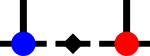SyTendense_rank.h File Reference

Calculates the numerical rank of a dense complex matrix. More...

#include "inc/dense/dense.h"Include dependency graph for dense_rank.h:This graph shows which files directly or indirectly include this file:

## Namespaces

syten
Syten namespace.

## Functions

double syten::full_rank_ness (DenseTensor< 2, double > &&a)
Estimates the full-rank-ness of a real matrix A (compared to its smaller dimension) using QR with pivoting. More...

double syten::full_rank_ness (DenseTensor< 2, double > const &a)
Estimates the full-rank-ness (compared to its smaller dimension) of a real matrix A using QR with pivoting. More...

double syten::full_rank_ness (DenseTensor< 2, std::complex< double > > &&a)
Estimates the full-rank-ness of a complex matrix A (compared to its smaller dimension) using QR with pivoting. More...

double syten::full_rank_ness (DenseTensor< 2, std::complex< double > > const &a)
Estimates the full-rank-ness (compared to its smaller dimension) of a complex matrix A using QR with pivoting. More...

Index syten::rank (DenseTensor< 2, double > &&a)
Estimates the rank of a real matrix A using QR with pivoting. More...

Index syten::rank (DenseTensor< 2, double > const &a)
Estimates the rank of a real matrix A using QR with pivoting. More...

Index syten::rank (DenseTensor< 2, std::complex< double > > &&a)
Estimates the rank of a complex matrix A using QR with pivoting. More...

Index syten::rank (DenseTensor< 2, std::complex< double > > const &a)
Estimates the rank of a complex matrix A using QR with pivoting. More...

## Detailed Description

Calculates the numerical rank of a dense complex matrix.